# Formula-driven graphics

There are several excellent graphics packages provided for R. The ggformula package currently builds on one of them, ggplot2, but provides a very different user interface for creating plots. The interface is based on formulas (much like the lattice interface) and the use of the chaining operator (%>%) to build more complex graphics from simpler components.

The ggformula graphics were designed with several user groups in mind:

• beginners who want to get started quickly and may find the syntax of ggplot2() a bit offputting,

• those familiar with lattice graphics, but wanting to be able to easily create multilayered plots,

• those who prefer a formula interface, perhaps because it is familiar from use with functions like lm() or from use of the mosaic package for numerical summaries.

## The basic formula template

The basic template for creating a plot with ggformula is

gf_plottype(formula, data = mydata)

or, equivalently,

mydata %>% gf_plottype(formula)

where

• plottype describes the type of plot (layer) desired (points, lines, a histogram, etc., etc.),

• mydata is a data frame containing the variables used in the plot, and

• formula describes how/where those variables are used.

For example, in a bivariate plot, formula will take the form y ~ x, where y is the name of a variable to be plotted on the y-axis and x is the name of a variable to be plotted on the x-axis. (It is also possible to use expressions that can be evaluated using variables in the data frame as well.)

The first form of the tempate is useful for simple plots or for multi-layered plots where different layers use different data. The second form is useful for multi-layered plots or plots with many arguments.

Here is a simple example:

library(ggformula)
gf_point(mpg ~ hp, data = mtcars)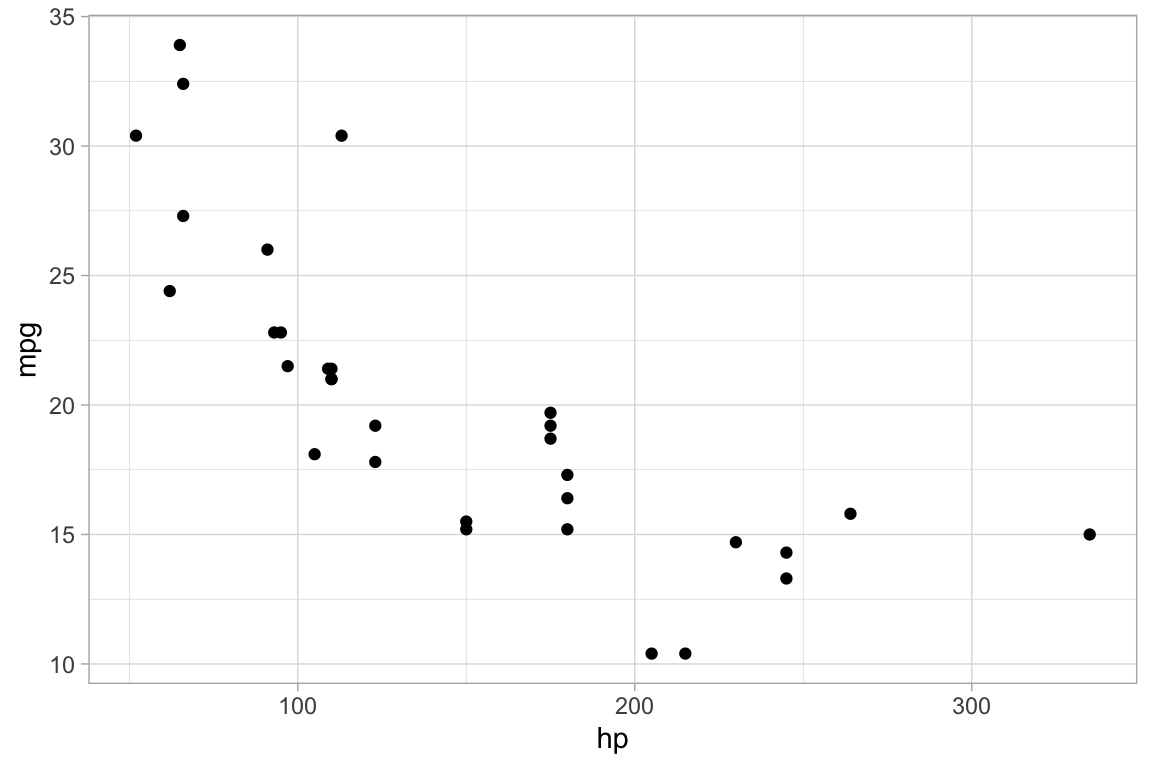mtcars %>% gf_point(mpg ~ hp)## Selecting the glyph type

The “kind of graphic” is specified by the name of the graphics function. All of the ggformula data graphics functions have names starting with gf_, which is intended to remind the user that they are formula-based interfaces to ggplot2: g for ggplot2 and f for “formula.” Commonly used functions include

• gf_point() for scatter plots
• gf_line() for line plots (connecting dots in a scatter plot)
• gf_density() or gf_dens() or gf_histogram() or gf_dhistogram() or gf_freqpoly() to display distributions of a quantitative variable
• gf_boxplot() or gf_violin() for comparing distributions side-by-side
• gf_counts() for bar-graph style depictions of counts.
• gf_bar() for more general bar-graph style graphics

The function names generally match a corresponding function name from ggplot2, although

• gf_counts() is a simplified special case of geom_bar(),
• gf_dens() is an alternative to gf_density() that displays the density plot slightly differently
• gf_dhistogram() produces a density histogram rather than a count histogram.

Each of the gf_ functions can create the coordinate axes and fill it in one operation. (In ggplot2 nomenclature, gf_ functions create a frame and add a layer, all in one operation.) This is what happens for the first gf_ function in a chain. For subsequent gf_ functions, new layers are added, each one “on top of” the previous layers.

## Attributes

Each of the marks in the plot is a glyph. Every glyph has graphical attributes (called aesthetics in ggplot2) that tell where and how to draw the glyph. In the above plot, the obvious attributes are x- and y-position:
We’ve told R to put mpg along the y-axis and hp along the x-asis, as is clear from the plot.

But each point also has other attributes, including color, shape, size, stroke, fill, and alpha (transparency). We didn’t specify those in our example, so gf_point() uses some default values for those – in this case smallish black filled-in circles.

### Specifying attributes

In the gf_ functions, you specify the non-position graphical attributes using additional arguments to the function. Attributes can be set to a constant value (e.g, set the color to “blue”; set the size to 2) or they can be mapped to a variable in the data or some expression involving the variables (e.g., map the color to sex, so sex determines the color groupings)

Attributes are set or mapped using additional arguments.

• adding an argument of the form attribute = value sets attribute to value.
• adding an argument of the form attribute = ~ expression maps attribute to expression

where attribute is one of color, shape, etc., value is a constant (e.g. "red" or 0.5, as appropriate), and expression may be some more general expression that can be computed using the variables in data (although often is is better to create a new variable in the data and to use that variable instead of an on-the-fly calculation within the plot).

The following plot, for instance,

• We use cyl to determine the color and carb to determine the size of each dot. Color and size are mapped to cyl and carb. A legend is provided to show us how the mapping is being done. (Later, we can use scales to control precisely how the mapping is done – which colors and sizes are used to represent which values of cyl and carb.)

• We also set the transparency to 50%. The gives the same value of alpha to all glyphs in this layer.

gf_point(mpg ~ hp, color = ~ cyl, size = ~ carb, alpha = 0.50, data = mtcars)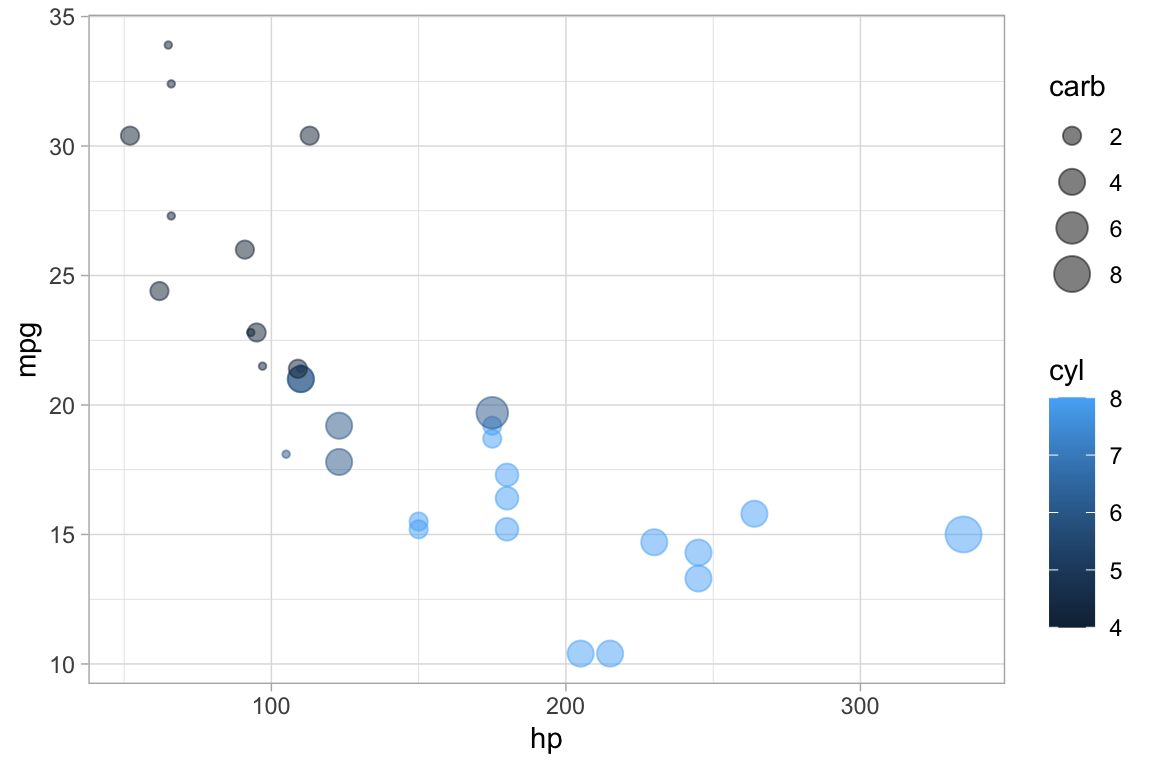### On-the-fly calculations

ggformula allows for on-the-fly calculations of attributes, although the default labeling of the plot is often better if we create a new variable in our data frame. In the examples below, since there are only three values for carb, it is easier to read the graph if we tell R to treat cyl as a categorical variable by converting to a factor (or to a string). Except for the labeling of the legend, these two plots are the same. In the second example, we see how the ggformula works well with data tranformations using %>%.

library(dplyr)
gf_point(mpg ~ hp,  color = ~ factor(cyl), size = ~ carb, alpha = 0.75, data = mtcars)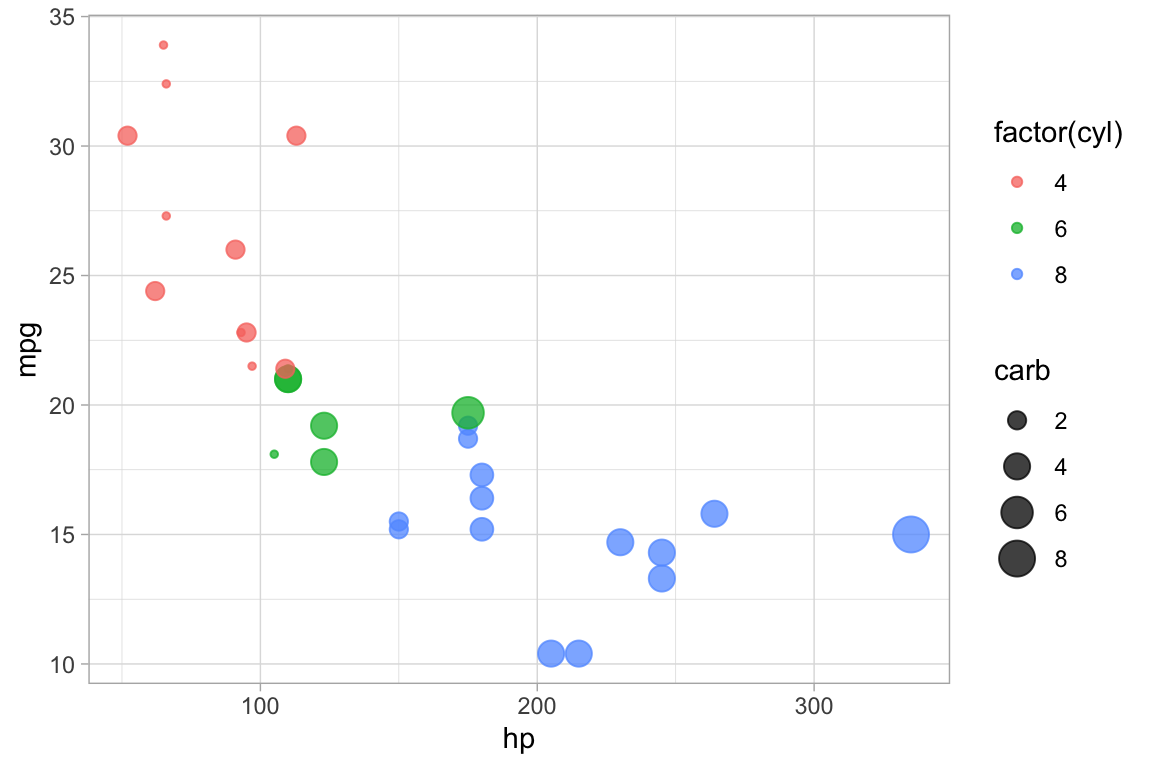mtcars %>%
mutate(cylinders = factor(cyl)) %>%
gf_point(mpg ~ hp,  color = ~ cylinders, size = ~ carb, alpha = 0.75)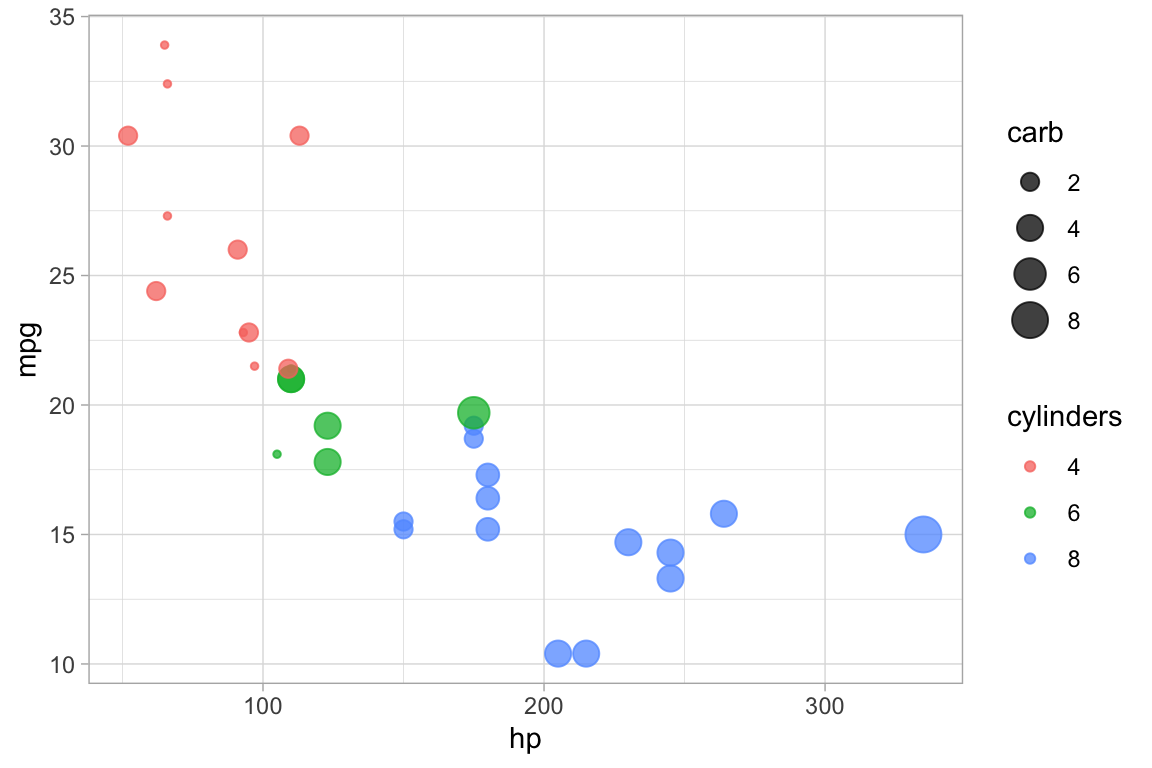## “One-variable” plots

For some plots, we only have to specify the x-position because the y-position is calculated from the x-values. Histograms, densityplots, and frequency polygons are examples. To illustrate, we’ll use density plots, but the same ideas apply to gf_histogram(), and gf_freqpolygon() as well. Note that in the one-variable density graphics, the variable whose density is to be calculated goes to the right of the tilde, in the position reserved for the x-axis variable.

data(penguins, package = "palmerpenguins")
gf_density( ~ bill_length_mm, data = penguins)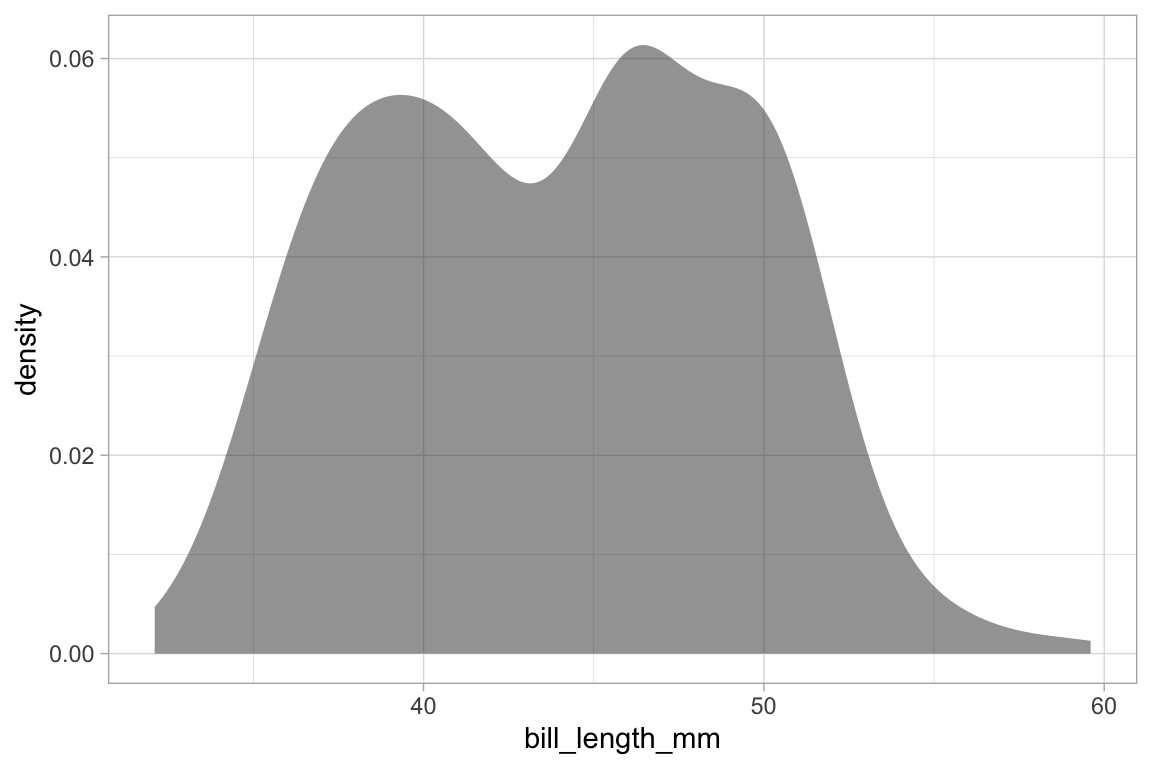gf_density( ~ bill_length_mm,  fill = ~ species,  alpha = 0.5, data = penguins)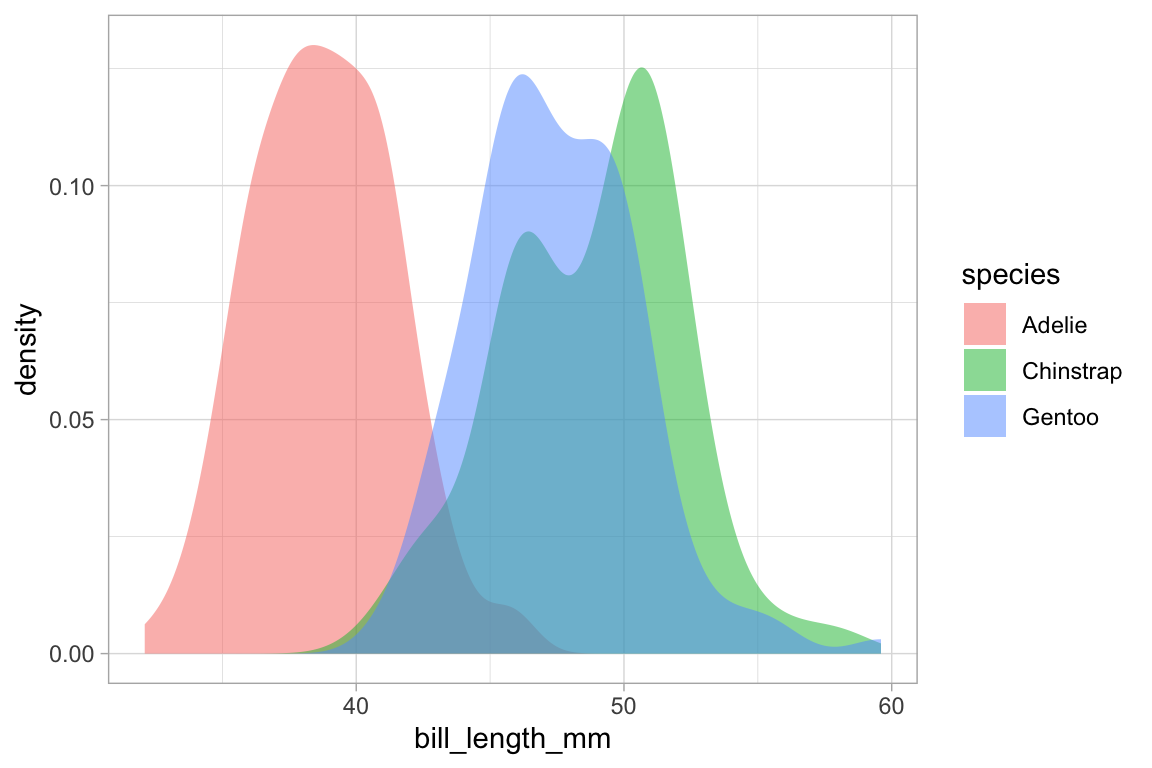# gf_dens() is similar, but there is no line at bottom/sides and the plot is not fillable
gf_dens( ~ bill_length_mm, color = ~ species,  alpha = 0.7, data = penguins)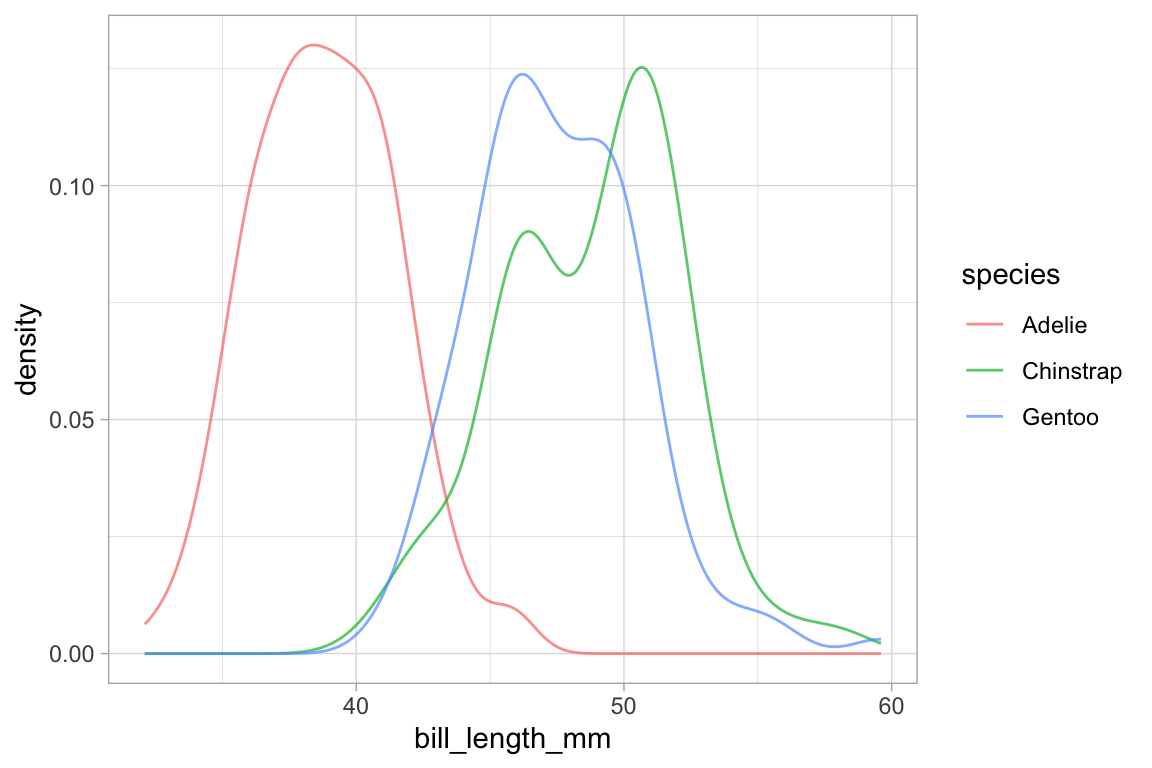# gf_dens2() is like gf_dens() but is fillable
gf_dens2( ~ bill_length_mm, fill = ~ species, data = penguins,
color = "gray50", alpha = 0.4)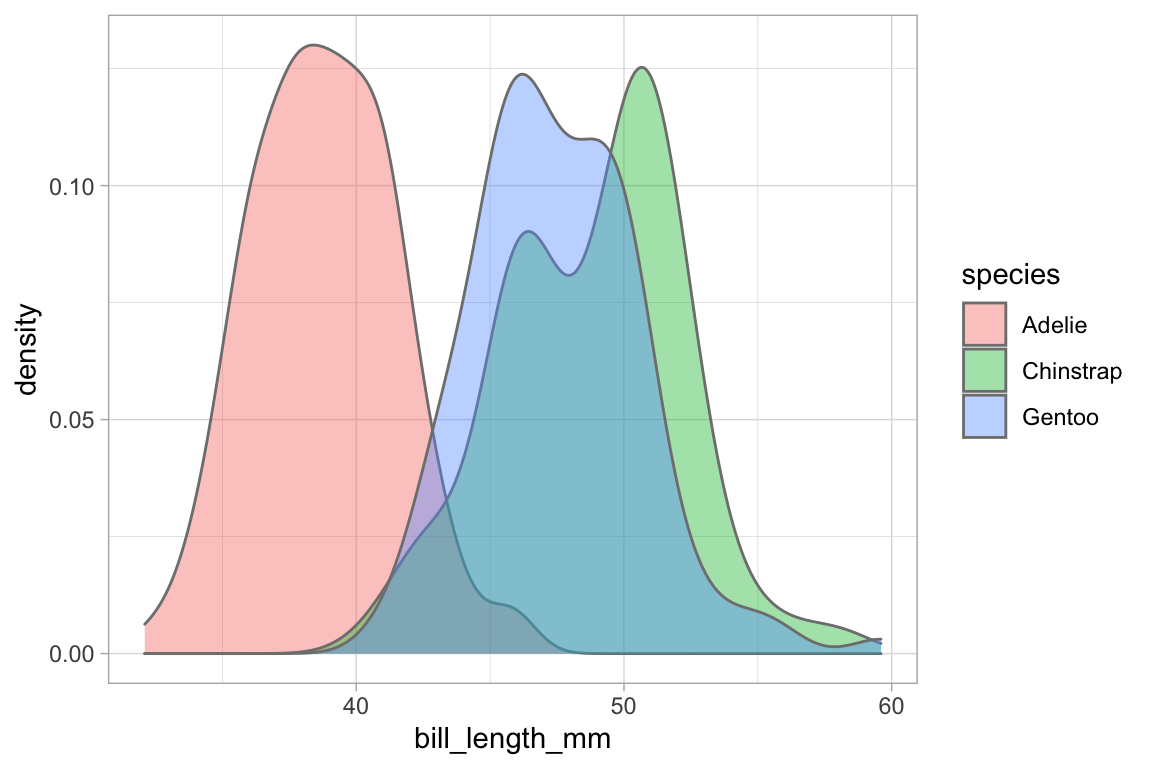Several of the plotting functions include additional arguments that do not modify attributes of individual glyphs but control some other aspect of the plot. In this case, adjust can be used to increase or decrease the amount of smoothing.

# less smoothing
penguins %>% gf_dens( ~ bill_length_mm, color = ~ species, alpha = 0.7, adjust = 0.25)  
## Warning: Removed 2 rows containing non-finite values (stat_density).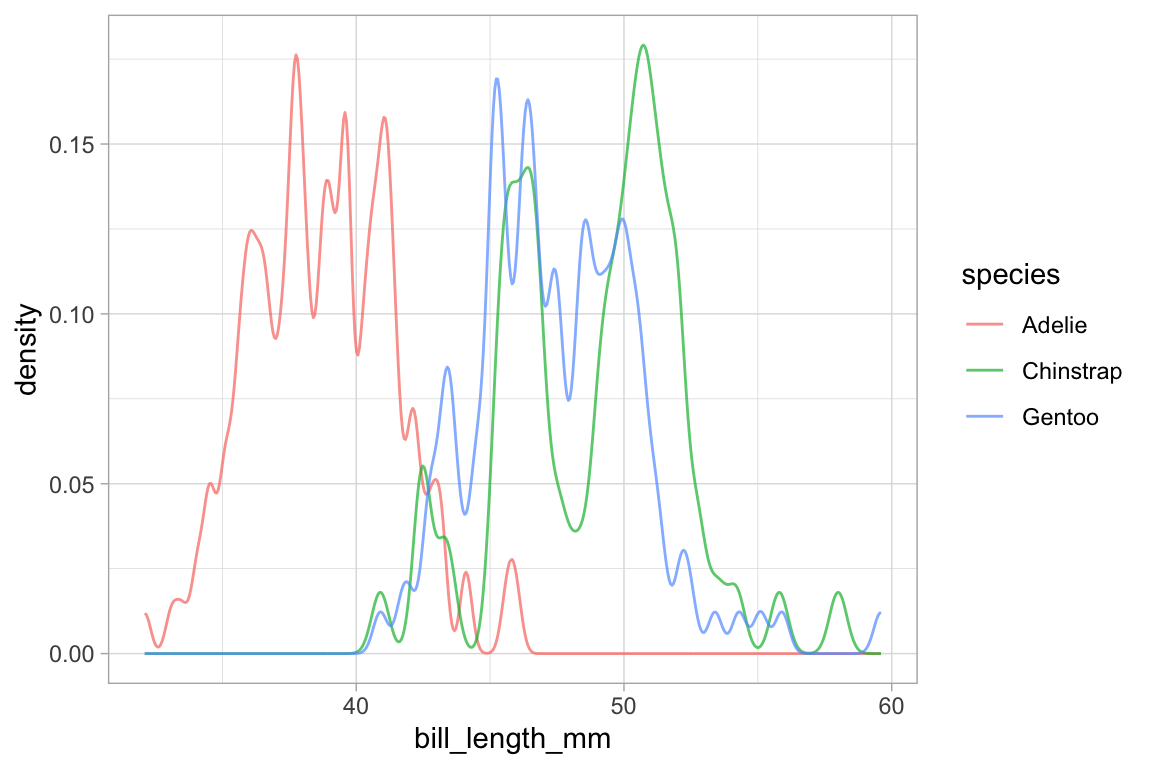# more smoothing
penguins %>% gf_dens( ~ bill_length_mm, color = ~ species, alpha = 0.7, adjust = 4)     
## Warning: Removed 2 rows containing non-finite values (stat_density).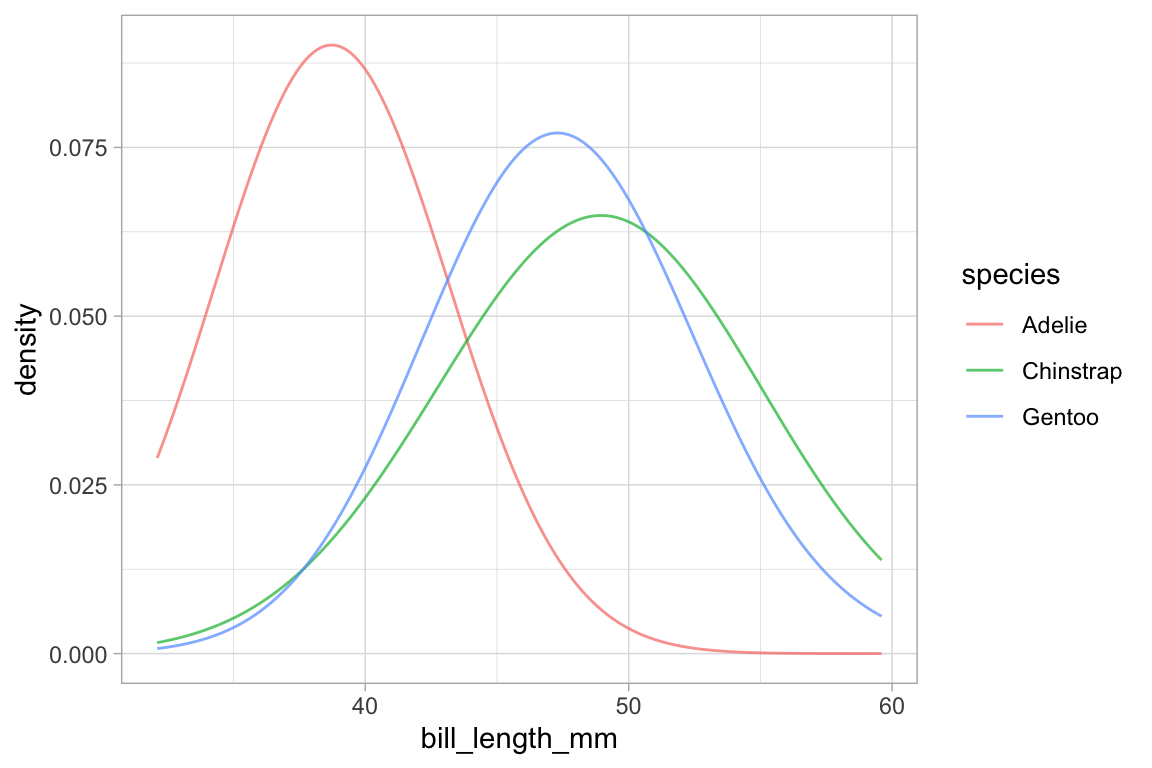## Learning more

To learn more about ggformula, see the longer version of this vignette available at https://projectmosaic.github.io/ggformula/. That version include sections on

• Adjusting position (stack, dodge, etc.)
• Faceting (coordinated subplots)
• Plot labeling (including using labels attached to the data by the labelled or expss packages)
• Chaining to create complext (multi-layer) plots
• Using jitter and transparency to deal with overplotting